# Calculation in Tableau: The concept of Addressing and Partitioning## Addressing and Partitioning

There are many complicated topics in Tableau that take a bit of effort to understand. One such is Addressing and Partitioning within a calculation in Tableau. Understanding this concept leads to more options to fine-tune table calculation’s scope, direction, and order of operations. Often, it is common to perform more than one table calculation at a time and defining the correct addressing and partitioning fields plays a vital role in those situations.

Partitions define the boundary values for the calculation. In other words, they define from where the calculation should restart. Addressing decides the direction the calculation should take. This is to say, they define the field on which the calculation has to happen.

By default, the table calculation in Tableau happens across the table. However, this changes while using Addressing.
On the other hand, any new dimension you add to the view is considered as a Partitioning field unless specified otherwise.

This blog illustrates the nuances of Addressing and Partitioning by creating 2 views that achieve the same result of ranking states according to sales within each region.
The first view is a dynamic view which you create in the current chart and the second view is a static view. The current data-set has sales of all the 49 US states from 2014 to 2017.

### Steps for creating a dynamic view using Calculation in Tableau

Step1:

Create a new Tableau project with Tableau Super-store data. In the new viz, create a calculated field named ‘index’.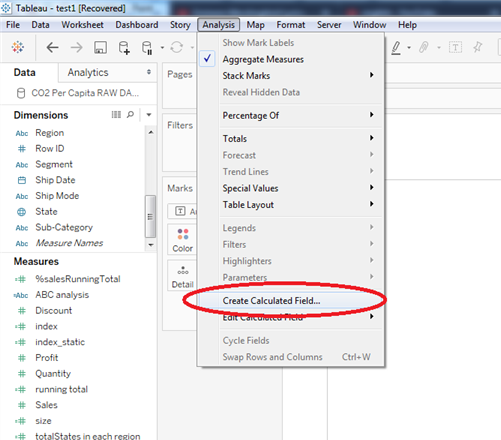Step 2:

Pull Region, and State to columns, Sales to rows, and index to text mark of the viz.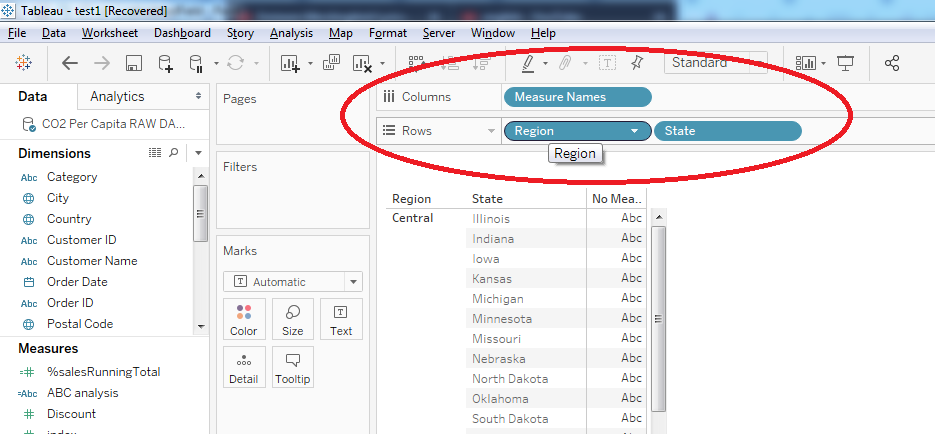Step 3:

By default, the index values are calculated across the table. In other words, the index value is reset for each row. Hence, the value is ‘1’ for all the States.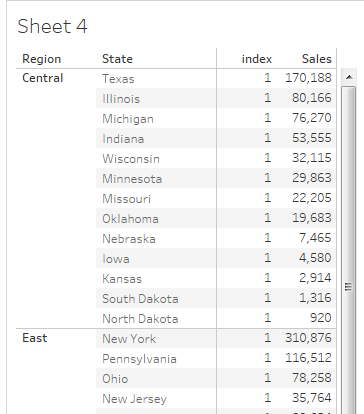But this isn’t of much use. However, states ranked within a region will certainly be of use. To get the desired result, edit the table calculation for the ‘index’ calculated field and choose the options as shown in step 4.

Step 4: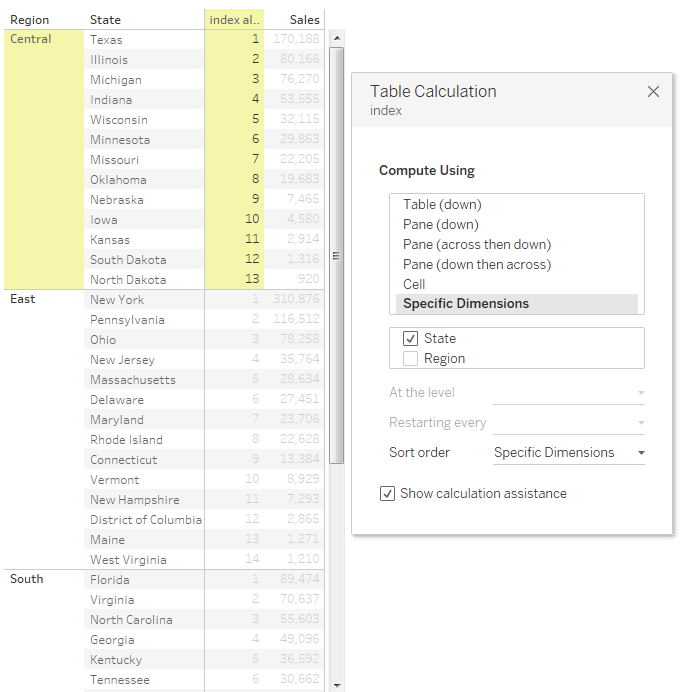Fig.1

In the above image, State is the Addressing field. Consequently, for each and every State, the calculation of the corresponding index value is done.  The other fields that you don’t choose become the Partitioning field. In other words, Region is the boundary for the index() calculation, so for every Region the calculation resets.

Now, you can achieve the same view without editing the table calculation in perspective, by following the steps below.

### Steps for creating a Static View using Calculation in Tableau

Step 1 :

Create a calculated field index_static, and edit the default table calculation as below.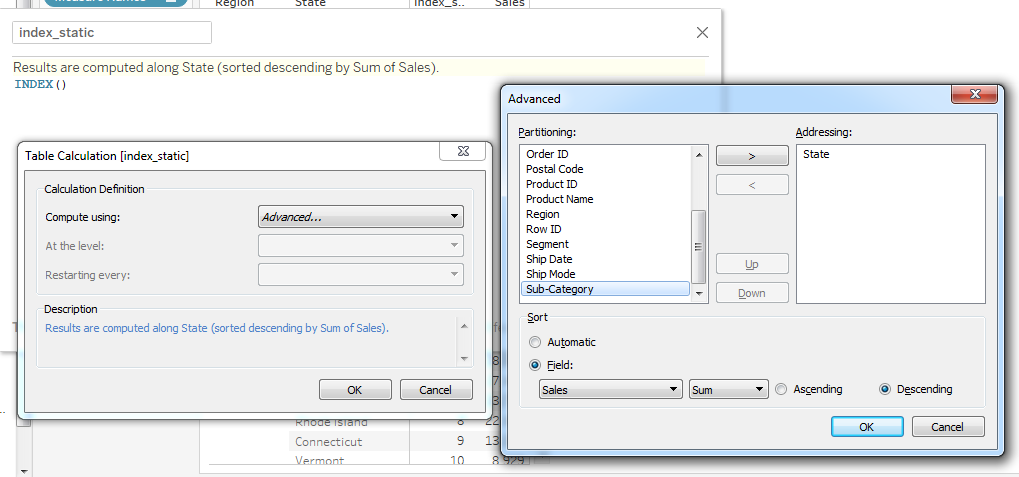Add the State to Addressing list, and sort them using Sales in the descending order.

Step 2:

Add Region, and State to columns, Sales to rows, and index_static to text mark of the viz.

Step 3:

The final result will be the same as Fig.1.

Addressing and Partitioning play a vital role in converting dynamic calculations to static.

As an example consider this: a dashboard has many levels of aggregation. The aggregate at one level needs to be at the next level. However, a current view can handle at most 2 levels of iteration. Therefore, to achieve the result, previous iterations have to move into table calculations. Defining the boundary and directions decides the accuracy of the calculation.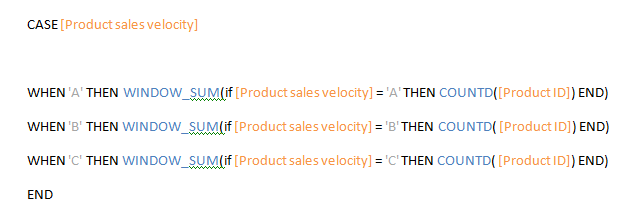The above chart categorizes the products into 3 buckets based on their sales value. There isn’t a direct way to obtain the count of products in each bucket from the view. A calculated field as shown above and proper setting of Addressing and Partitioning fields will fetch the count required.

To reiterate the definition, Partitioning is defining the scope of the table calculation and Addressing answers the question “apply the calculation on what?”.

Please share your comments and watch this blog space for more Tableau related blog posts